### Home > PC > Chapter 8 > Lesson 8.2.5 > Problem8-142

8-142.
1. Given the sequence defined by an+1 =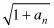where a1 = 1. What is the limit of the sequence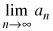? Homework Help ✎

1. Using the process as described in problem 8-135, estimate the limit.

2. We can find the limit using algebra by solving the equation L =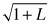. Solve the equation to find the exact value of the limit.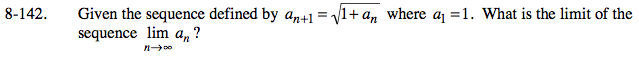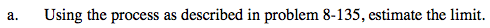a5 =
a6 =
a7 =
a8 =
a9 =
a10 =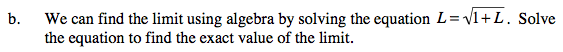L2 = 1 + L
L2L − 1 = 0